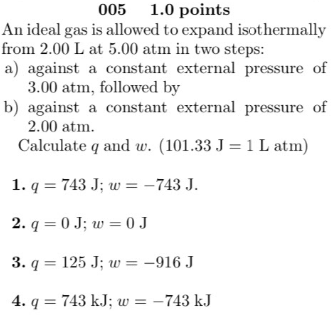# An ideal gas is allowed to expand isothermally from 2.00 L at 5.00 atm in two steps; a) against a constant external pressure of 3.00 atm, followed by b) against a constant external pressure of 2.00 atm. Calculate q and w. (1.1.33 J = 1 L atm) 1. q = 743 J; w = -743 J. 2. q = 0 J; w = 0 J. 3. q = 125 J; w = -916 J. 4. q = 743 kJ; w = -743 kJ.